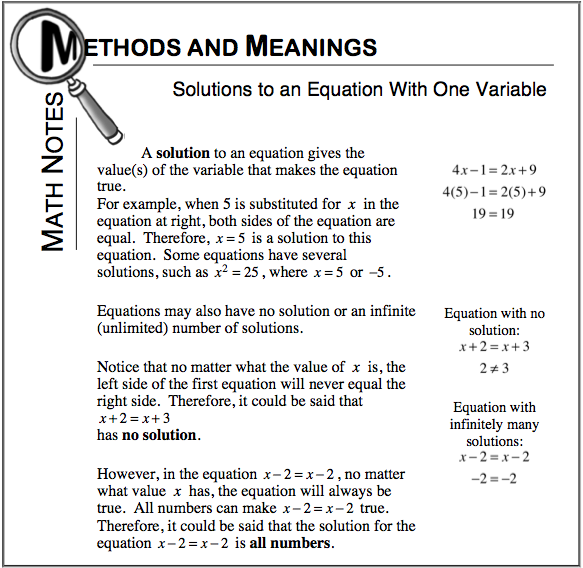Home > CC2MN > Chapter cc26 > Lesson cc26.2.6 > Problem6-122

6-122.

Simplify and solve each equation below for $x$. Show your work and record your final answer. Homework Help ✎

1. $24+2x=3x+2(3·4)$

Use the Order of Operations to simplify the equation.

$24+2x=3x+2(3·4)\\ 24+2x=3x+2(12)\\ 24+2x=3x+24$

Start isolating the variable by subtracting $24$ from each side of the equation.

$\quad 24+2x=3x+24\\ -24\qquad \qquad \ \ \ -24\\ \qquad \quad 2x=3x$

Continue to isolate the variable by subtracting $2x$ from both sides.

$\quad 2x=3x\\ -2x \ -2x\\ \quad \ \ 0=x$

$x=0$

2. $24+3x=3x+3(7-1)$

Follow the method of isolating the variable as presented in part (a).

3. $2(12+x)=2x+24$

Follow the method of isolating the variable as presented in part (a). Use the Math Notes box below for help.